Courses

# The Direct Stiffness Method: Beams - 7 GATE Notes | EduRev

## GATE : The Direct Stiffness Method: Beams - 7 GATE Notes | EduRev

The document The Direct Stiffness Method: Beams - 7 GATE Notes | EduRev is a part of the GATE Course Structural Analysis.
All you need of GATE at this link: GATE

Example 29.2
A continuous beam ABCD is carrying a uniformly distributed load of 5 kN / m as shown in Fig. 29.4a. Compute reactions due to following support settlements.

Support B 0.005 m vertically down wards.

Support C 0.010 m vertically down wards.

Assume E = 200 GPa and I = 4 x 10-4 m4.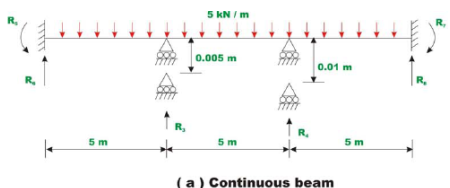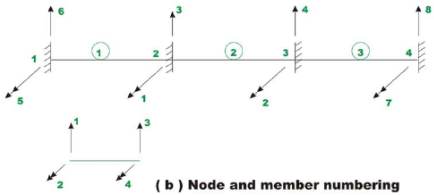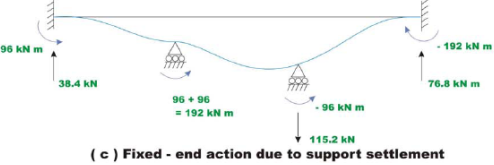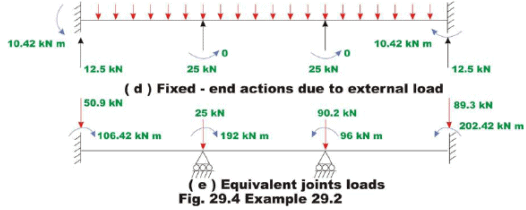Solution
The node and member numbering are shown in Fig. 29.4(b), wherein the continuous beam is divided into three beam elements. It is observed from the figure that the unconstrained degrees of freedom are u1 and u2 . The fixed end actions due to support settlements are shown in Fig. 29.4(c). and fixed end moments due to external loads are shown in Fig. 29.4(d). The equivalent joint loads due to support settlement and external loading are shown in Fig. 29.4(e). The fixed end actions due to support settlement are,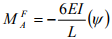where ψ is the chord rotation and is taken + ve if the rotation is counterclockwise.

Substituting the appropriate values in the above equation,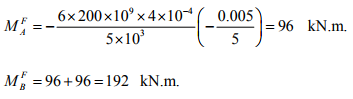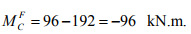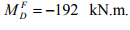(1)

The vertical reactions are calculated from equations of equilibrium. The fixed end actions due to external loading are,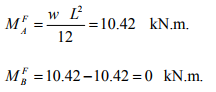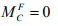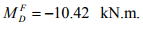(2)

In the next step, construct member stiffness matrix for each member.

Member 1, L = 5m , node points 1-2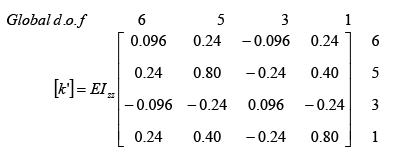(3)

Member 2, L = 5 m , node points 2-3.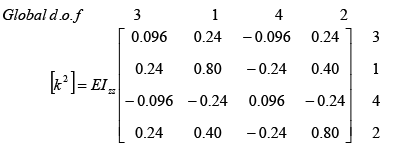(4)

Member 3, L = 5 m , node points 3-4.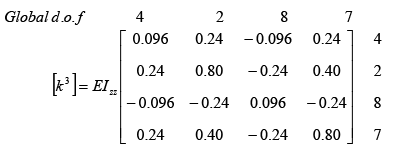(5)

On the member stiffness matrix, the corresponding global degrees of freedom are indicated to facilitate assembling. The assembled global stiffness matrix is of the order 8×8. Assembled stiffness matrix [K]is,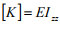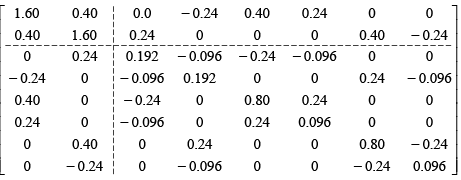(6)

The global load vector corresponding to unconstrained degree of freedom is,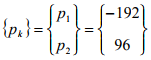(7)

Writing the load displacement relation for the entire continuous beam,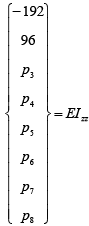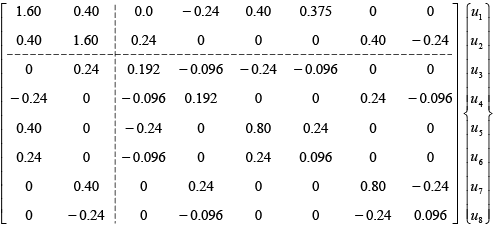(8)

We know that u3 = u4 = u5 = u6 = u7 = u8 = 0 . Thus solving for unknowns displacements u1 and u2 from equation,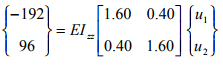(9)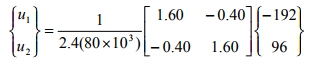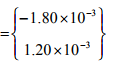(10)

u1 =-1.80 x 10-3 radians;    u2 = 1.20 x 10-3 radians                         (11)

The unknown joint loads are calculated as,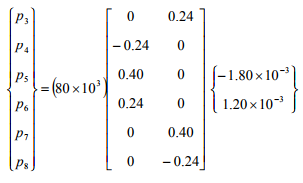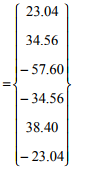(12)

Now the actual support reactions R3 , R4 , R5 , R6 , R7 and R8 must include the fixed end support reactions. Thus,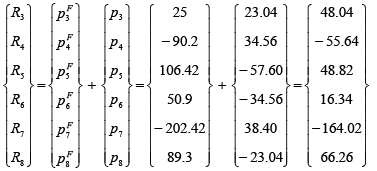(13)

R3 = 48.04 kN; R4 = -55.64 kN;    R5 = 48.82 kN.m;
R6 = 16.34 kN; R7 = -164.02 kN.m; R8 = 66.26 kN                               (14)

Summary

The effect of temperature changes and support settlements can easily be incorporated in the direct stiffness method and is discussed in the present lesson. Both temperature changes and support settlements induce fixed end actions in the restrained beams. These fixed end forces are handled in the same way as those due to loads on the members in the analysis. In other words, the global load vector is formulated by considering fixed end actions due to both support settlements and external loads. At the end, a few problems are solved to illustrate the procedure.

Offer running on EduRev: Apply code STAYHOME200 to get INR 200 off on our premium plan EduRev Infinity!

## Structural Analysis

30 videos|122 docs|28 tests

,

,

,

,

,

,

,

,

,

,

,

,

,

,

,

,

,

,

,

,

,

;